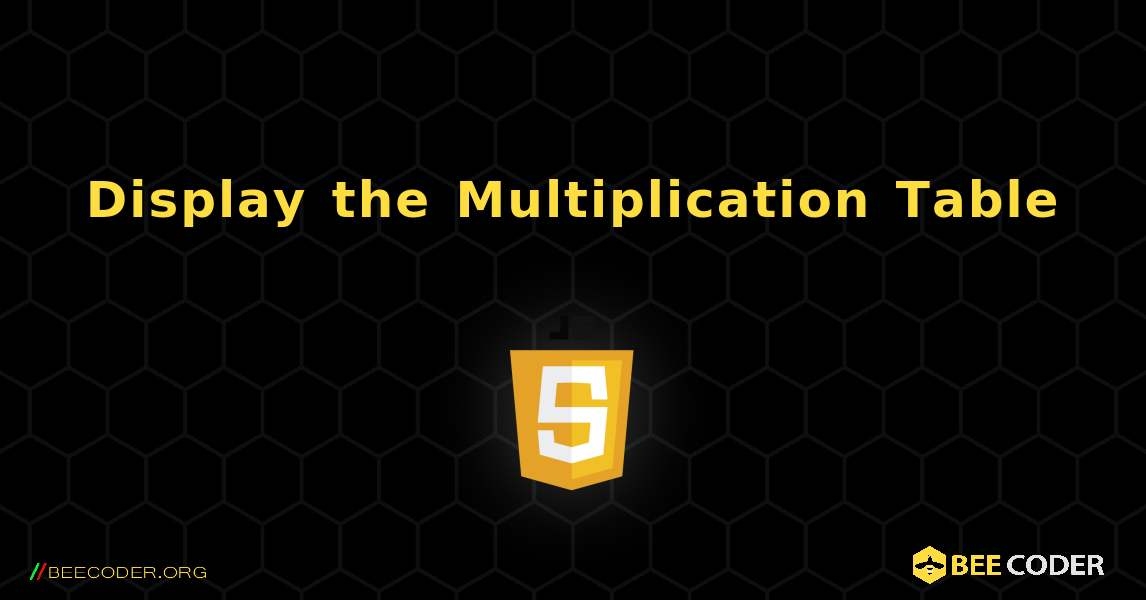# Display the Multiplication Table. JavaScriptJavaScript

Output result
```Enter an integer: 3
3 * 1 = 3
3 * 2 = 6
3 * 3 = 9
3 * 4 = 12
3 * 5 = 15
3 * 6 = 18
3 * 7 = 21
3 * 8 = 24
3 * 9 = 27
3 * 10 = 30```## BeeCoder.ORG

###### open source code and examples

We have been online since 2021 and 1 millions of people around the globe have visited our website since then

More visitors every month